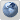F~u~c~k i~t！
Would you like to react to this message? Create an account in a few clicks or log in to continue.
Top posting users this month
 No user

GeoMap

# Now this forum support MathJAX $$LaTex$$

2 posters##Now this forum support MathJAX (LaTex)

You can use '\$\$' '\$\$' or escaped '[' ']' to enter display math, and use escaped '(' ')' to enter inline latex equation:
$$e=mc^2$$

Good! $$\alpha=\beta+\gamma$$

Here is a good example:
[You must be registered and logged in to see this link.]##Re: Now this forum support MathJAX $$LaTex$$

Quite interesting, try to plot this:$x = 3y\log y - \frac{1}{{36}}\exp \left[ { - {{\left( {36y - \frac{{36}}{e}} \right)}^4}} \right]$

$x = 3y\log y - \frac{1}{{36}}\exp \left[ { - {{\left( {36y - \frac{{36}}{e}} \right)}^4}} \right]$

$$x = 3y\log y - {1 \over {36}}\exp \left[ { - {{\left( {36y - {{36} \over e}} \right)}^4}} \right]$$halalaPermissions in this forum:
You cannot reply to topics in this forum